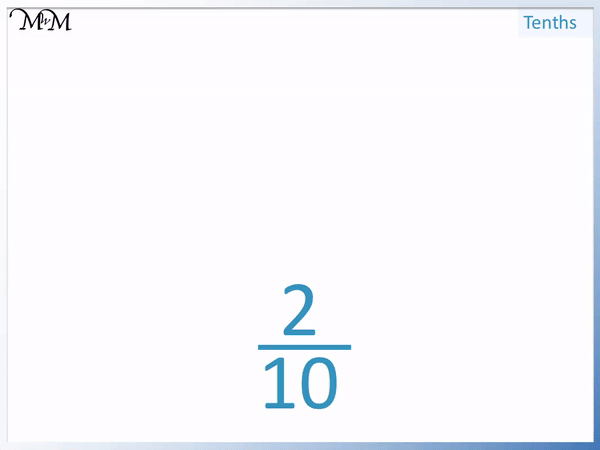# Counting in Tenths

Counting in Tenths• The fraction one tenth is written as   1 / 10  .
• This means 1 divided by 10.
• We take one whole and divide it into ten equal parts.
• Ten tenths make   10 / 10  .
• Ten tenths make a whole.

One tenth is written as   1 / 10  .

Ten tenths make one whole.• The number on the top of the fraction tells us how many tenths we have.
•   5 / 10   is half way between 0 and 1.
•   10 / 10   is one whole.Supporting Lessons

# Counting in Tenths

## What are Tenths?

A tenth is a fraction written as   1 / 10  .

The 1 on the top of the fraction means to start with one whole.

The line in the fraction means to divide.

Then we have a 10 on the bottom of the fraction so we divide by 10.One tenth means one whole divide into 10 equal parts.

Here we have 2 tenths, which is written as   2 / 10  .

It means to take 2 wholes and divide them both by 10.When we divide each whole by 10, we get a tenth.

We have 2 tenths.

## Counting in Tenths

We can count up in tenths by increasing the number on the top of the fraction.

The number on the bottom of these fractions tells us how many tenths we have.

As the number goes up, we add another tenth.10 tenths is written as   10 / 10  , which is one whole.

10 / 10   means 10 divided by 10, which equals one.

We can count up in tenths on a number line from 0 until we reach 1.We can see that   5 / 10   is half way and so   5 / 10   is one half.

10 / 10   is 10 tenths, which is the same as 1 on the number line.Now try our lesson on Shading Fractions of Shapes where we learn how to shade a fraction.error: Content is protected !!